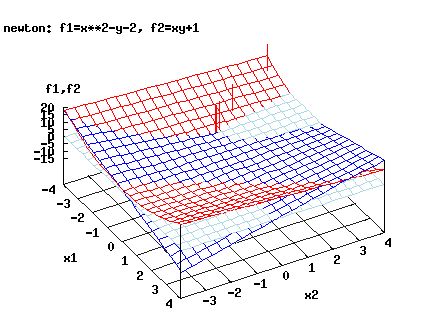## ZERO -- Zeros of Functions and Systems

The following table shows performance of Newtons method for the problem f1=x**2-y-2=0 and f2=x*y+1=0 with initial guess -4,4

 x1 x2 f1 f2 ||inv(jacob)|| -4.0000 4.0000 10.000 -15.000 .33333 -2.4722 1.7778 2.3341 -3.3951 .48011 -1.8176 .87522 .42851 -.59082 .60279 -1.6346 .63831 .33506E-01 -.43366E-01 .65321 -1.6182 .61819 .26912E-03 -.33013E-03 .65832

See also the picture. The solutions of the system occur where the three colors corresponding to the surfaces z=f1(x,y), z=f2(x,y) and z=0 (red, dark blue and light blue) meet. The iterates are represented by vertical bars.Single Variable

 MANBIS Package for Locating and Computing Efficiently Many Roots of a Function (C++) f77-zero,C++ version,C version f is a function of one real variable (Brent-Decker method), using function values only, zerofinder , needs inclusion by change of sign on interval SERVER server routines for brent.shar (C) polyzero f is a polynomial of one complex variable and has real coefficients (least squares method, interactive/file input, f77/C) MultRoot f polynomial with real (inexact) coefficients, especially for multiple roots (Matlab) companionC-version f polynomial with real coefficients, QR for eigenvalues of companion matrix AberthC-version f is a polynomial with complex coefficients MPSolve multiple precision, complex coefficients, f77/f90 MullerC-version f is a general function of one complex variable DFZERO search for a zero in a given interval RPOLY find all zeroes of a real polynomial (Jenkins-Traub method) f90-version, C++-version, Javascript for RPOLY Madsen find all zeroes of a real polynomial, C++ version CPOLY find all zeroes of a complex polynomial (Jenkins-Traub method) f90-version, C-version, C++ version RootFinder find all zeroes of a complex polynomial (several methods, root refinement, Windows version, web-based version) Polynomial Equation Solver low degree algebraic solver and Jenkins-Traub, real and complex, various languages RROOT safely enclosing zeroes of a continuous function in an interval

Multiple Variable

 MULTIPLICITY Matlab software for computing multiplicity structure of nonlinear systems, paper RATIONAL Four different rational solvers for sparse and dense LINEAR SYSTEMS using GMP, large test set (C) KINSOL part of the SUNDIALS suite for ODE/DAE (C, Fortran interface) ALIAS Solve systems of equations or inequalities with interval arithmetic (C++) TRESNEI Solve systems of equations or inequalities with least squares approach (Matlab) box_zeros f depends on one or more complex variables, zeros sought in box (based on TOMS666) HYBRJ f not too nonlinear, exact Jacobian (Powell's hybrid method) HYBRD f90 version not too nonlinear, Powells hybrid method, finite difference Jacobian DogLeg Powell's dogleg method (Matlab) csolve Robust solver by C. Sims (Matlab) STRSCNE Trust region method for bound-constrained nonlinear systems (Matlab) CoDoSol Constrained dogleg method for nonlinear systems with simple bounds(Matlab) CRBond's code real/complex one/multidimensional rootfinders and LS solvers (C/C++) Filtrane part of Galahad, nonlinear constraint solver, filter method, f2003 PITCON f not too nonlinear, no good initial guess available, continuation method LOCA Library of continuation algorithms; uses bordering algorithm to permit very large dimensions; distributed memory parallel (C), part of Trilinos Continuation Toolbox MatCont Includes continuation of limit cycles and codim 1 bifurcations (Matlab) AUTO Another Matlab continuation toolbox (for dynamical systems) SNES part of PETSC , serial and MPI parallel versions SALSA Self-Adapting Large-scale Solver Architecture for linear and nonlinear systems PEQN/PEQL inexact Newton and inverse column update methods, for large/sparse problems nnes zeros sought in box: l<=x<=u, see also users' guide DAFNE differential equations approach with second order system inspired by mechanics CHABIS characteristic bisection method BERTINI Solving polynomial systems by homotopy continuation (C) Bertini-Lab Solving polynomial systems by homotopy continuation (Matlab) EPSILON Maple-based package for operations on polynomials including solving systems HOMPACK globally convergent continuation method for polynomial systems HOMPACK90 f90 version of HOMPACK POLSYS_PLP more sophisticated version of HOMPACK90 TENSOLVE A Software Package for Solving Systems of Nonlinear Equations and Nonlinear Least-squares Problems Using Tensor Methods f90 version toms/795, PHCPACK A general-purpose solver for polynomial systems by homotopy continuation INTBIS interval method, f77

(Constrained) minimization approach

Rootfinders based on Newtons method or its modification will run into considerable trouble when presented with a problem where singularities of the Jacobian occur. In this case the use of unconstrained or constrained minimization might help, since there exist minimization methods which are rather robust if the constraint gradients are linearly dependent. To use an unconstrained minimizer, choose e.g. f(x)=||F(X)||^2 (the Euclidean length squared) and minimize this with some code proposed in the unconstrained minimization section. To use a constrained minimizer, choose simply f==0 as an objective function and minimize this under the constraint F(x)=0. The following code works often successfully in this situation:

 LANCELOT A or LANCELOT B augmented Lagrangian based method